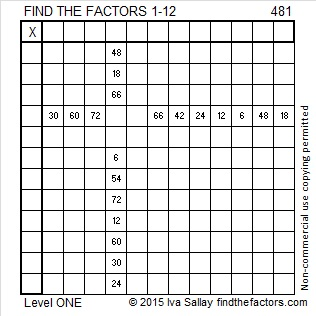# 481 and Level 1

Can you find the greatest common factor of the three numbers in this Pythagorean triple 156-455-481? Or in Pythagorean triple 185-444-481?

481 is also the hypotenuse in two primitive Pythagorean triples: 319-360-481 and 31-480-481. Since they are primitive triples, their greatest common factor is 1.Print the puzzles or type the solution on this excel file: 12 Factors 2015-05-04

—————————————————————————————————

• 481 is a composite number.
• Prime factorization: 481 = 13 x 37
• The exponents in the prime factorization are 1 and 1. Adding one to each and multiplying we get (1 + 1)(1 + 1) = 2 x 2 = 4. Therefore 481 has exactly 4 factors.
• Factors of 481: 1, 13, 37, 481
• Factor pairs: 481 = 1 x 481 or 13 x 37
• 481 has no square factors that allow its square root to be simplified. √481 ≈ 21.93171—————————————————————————————————